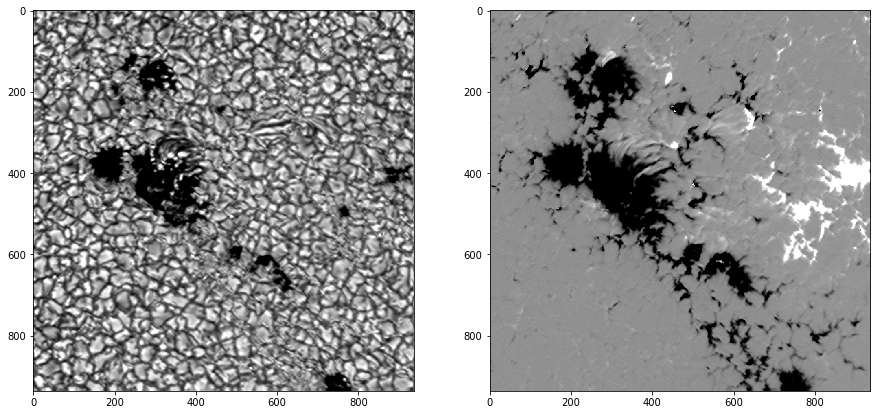# Feature detection with scikit-image

## Feature detection and analysis¶

### In this project I will be using the powerful image analysis package skimage to segment objects in an image of interest and measure properties of detected features.¶

#### I will be using an example, a brightness image at the continuum around 500 nm of an active region imaged by Sunrise balloon observatory through the spectropolarimeter IMAX. The aim is to find the small-scale magnetic elements and inspect their feature properties.¶

In :
```## loading libraries
import matplotlib.pyplot as plt
import numpy as np
import pyfits
from numpy import flipud
```
In :
```## loading data
p = '/home/fatima/Desktop/project_2/alignment/'
fig = plt.figure(figsize=(15,10))
ax1.imshow(imax, cmap='gray',vmin=imax.mean()-2*imax.std(),vmax = imax.mean()+2*imax.std())
ax2.imshow(B, cmap='gray',vmin=B.mean()-2*B.std(),vmax = B.mean()+2*B.std())
```
Out:
`<matplotlib.image.AxesImage at 0x7fc0aaae9d10>`#### A pixel by pixel scatter plot of the brightness vs magnetic field allows me to identify the region where the magnetic elements belong to. They are all the pixels with a magnetic field above 600 G. I will make a contour plot enclosing all pixels having this threshold.¶

In :
```x = np.arange(imax.shape)
y = np.arange(imax.shape)
x,y = np.meshgrid(x,y)        #making a meshgrid with the dimensions of imax image
```
In :
```fig = plt.figure(figsize=(15,10))
`<matplotlib.contour.QuadContourSet at 0x7fc0aa9b60d0>`